Next: About this document ... Up: lab_template Previous: lab_template

Subsections

# MA 1024 Lab 4: Partial derivatives, directional derivatives, and the gradient

## Purpose

The purpose of this lab is to acquaint you with using Maple to compute partial derivatives, directional derivatives, and the gradient.

## Getting Started

To assist you, there is a worksheet associated with this lab that contains examples. You can copy that worksheet to your home directory with the following command, which must be run in a terminal window, not in Maple.

cp ~bfarr/Pardiff_start.mws ~


You can copy the worksheet now, but you should read through the lab before you load it into Maple. Once you have read to the exercises, start up Maple, load the worksheet Pardiff_start.mws, and go through it carefully. Then you can start working on the exercises.

## Background

For a function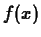of a single real variable, the derivative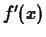gives information on whether the graph of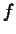is increasing or decreasing. Finding where the derivative is zero was important in finding extreme values. For a function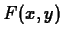of two (or more) variables, the situation is more complicated.

### Partial derivatives

A differentiable function,, of two variables has two partial derivatives:and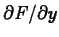. As you have learned in class, computing partial derivatives is very much like computing regular derivatives. The main difference is that when you are computing, you must treat the variable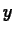as if it was a constant and vice-versa when computing.

The Maple commands for computing partial derivatives are D and diff. The Getting Started worksheet has examples of how to use these commands to compute partial derivatives.

### Directional derivatives

The partial derivativesandof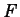can be thought of as the rate of change ofin the direction parallel to the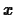andaxes, respectively. The directional derivative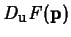, where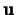is a unit vector, is the rate of change ofin the direction. There are several different ways that the directional derivative can be computed. The method most often used for hand calculation relies on the gradient, which will be described below. It is also possible to simply use the definition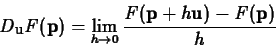to compute the directional derivative. However, the following computation, based on the definition, is often simpler to use.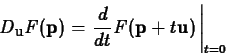One way to think about this that can be helpful in understanding directional derivatives is to realize thatis a straight line in the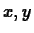plane. The plane perpendicular to theplane that contains this straight line intersects the surface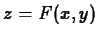in a curve whosecoordinate is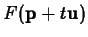. The derivative ofatis the rate of change ofat the point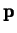moving in the direction.

Maple doesn't have a simple command for computing directional derivatives. There is a command in the tensor package that can be used, but it is a little confusing unless you know something about tensors. Fortunately, the method described above and the method using the gradient described below are both easy to implement in Maple. Examples are given in the Getting Started worksheet.

### The Gradient

The gradient of, written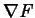, is most easily computed as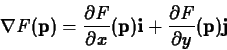As described in the text, the gradient has several important properties, including the following.
• The gradient can be used to compute the directional derivative as follows.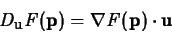• The gradient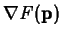points in the direction of maximum increase of the value ofat.
• The gradientis perpendicular to the level curve ofthat passes through the point.
• The gradient can be easily generalized to apply to functions of three or more variables.

Maple has a fairly simple command grad in the linalg package (which we used for curve computations). Examples of computing gradients, using the gradient to compute directional derivatives, and plotting the gradient field are all in the Getting Started worksheet.

## Exercises

1. Compute the three distinct second order partial derivatives of the functionYou may use either the D or diff commands or both, as you wish.

2. Consider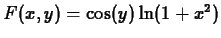. Compute the directional derivative ofat the point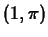in the direction of the vector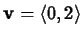using each of the two methods demonstrated in the Getting Started worksheet. Maple will probably not simplify the answer unless you tell it to. Use either the value command or the simplify command to show that the directional derivative is zero. Explain this answer geometrically in terms of the vector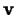and the contour ofthat passes through the point.

3. One of the two functions below has a minimum at the origin and the other has what you will learn is called a saddle point at the origin. Using the technique demonstrated in the Getting Started worksheet, plot the gradient field and a contour plot on the same graph for each function. (That is, one combined plot for each function.) Which plot (contour plot or gradient field plot) do you think gives you more information about the behavior of the function? Is your answer the same for both functions? Please explain.

1.2.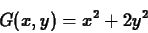Next: About this document ... Up: lab_template Previous: lab_template
Dina Solitro
2001-04-10